# Meta-Analytic-Predictive Priors for Variances

#### 2022-08-08

Applying the meta-analytic-predictive (MAP) prior approach to historical data on variances has been suggested in . The utility is a better informed planning of future trials which use a normal endpoint. For these reliable information on the sampling standard deviation is crucial for planning the trial.

Under a normal sampling distribution the (standard) unbiased variance estimator for a sample $$y_j$$ of size $$n_j$$ is

$s^2_j = \frac{1}{n_j-1} \sum_{i=1}^{n_j} (y_{j,i} - \bar{y}_j)^2,$

which follows a $$\chi^2_\nu$$ distribution with $$\nu_j = n_j-1$$ degrees of freedom. The $$\chi^2_\nu$$ can be rewritten as a $$\Gamma$$ distribution

$s^2_j|\nu_j,\sigma_j \sim \Gamma(\nu_j/2, \nu_j/(2\,\sigma^2_j))$ $\Leftrightarrow s^2_j \, \nu_j /2 |\nu_j,\sigma_j \sim \Gamma(\nu_j/2, 1/\sigma^2_j),$

where $$\sigma_j$$ is the (unknown) sampling standard deviation for the data $$y_j$$.

While this is not directly supported in RBesT, a normal approximation of the $$\log$$ transformed $$\Gamma$$ variate can be applied. When $$\log$$ transforming a $$\Gamma(\alpha,\beta)$$ variate it’s moment and variance can analytically be shown to be (see , for example)

$E[\log(X)] = \psi(\alpha) - \log(\beta)$ $Var[\log(X)] = \psi^{(1)}(\alpha).$

Here, $$\psi(x)$$ is the digamma function and $$\psi^{(1)}(x)$$ is the polygamma function of order 1 (second derivative of the $$\log$$ of the $$\Gamma$$ function).

Thus, by approximating the $$\log$$ transformed $$\Gamma$$ distribution with a normal approximation, we can apply gMAP as if we were using a normal endpoint. Specifically, we apply the transform $$Y_j=\log(s^2_j \, \nu_j /2) - \psi(\nu_j/2)$$ such that the meta-analytic model directly considers $$\log \sigma_j$$ as random variate. The normal approximation becomes more accurate, the larger the degrees of freedom are. The section at the bottom of this vignette discusses this approximation accuracy and concludes that independent of the true $$\sigma$$ value for 10 observations the approxmation is useful and a very good one for more than 20 observations.

In the following we reanalyze the main example of reference  which is shown in table 2:

study sd df
1 12.11 597
2 10.97 60
3 10.94 548
4 9.41 307
5 10.97 906
6 10.95 903

Using the above equations (and using plug-in estimates for $$\sigma_j$$) this translates into an approximate normal distribution for the $$\log$$ variance as:

hdata <- mutate(hdata,
alpha=df/2,
beta=alpha/sd^2,
logvar_mean=log(sd^2 * alpha) - digamma(alpha),
logvar_var=psigamma(alpha,1))
study sd df alpha beta logvar_mean logvar_var
1 12.11 597 298.5 2.0354 4.9897 0.0034
2 10.97 60 30.0 0.2493 4.8071 0.0339
3 10.94 548 274.0 2.2894 4.7867 0.0037
4 9.41 307 153.5 1.7335 4.4868 0.0065
5 10.97 906 453.0 3.7643 4.7914 0.0022
6 10.95 903 451.5 3.7656 4.7878 0.0022

In order to run the MAP analysis a prior for the heterogeniety parameter $$\tau$$ and the intercept $$\beta$$ is needed. In reference  it is demonstrated that the (approximate) sampling standard deviation of the $$\log$$ variance is $$\sqrt{2}$$. Thus, a HalfNormal(0,sqrt(2)/2) is a very conservative choice for the between-study heterogeniety parameter. A less conservative choice is HalfNormal(0,sqrt(2)/4), which gives very similar results in this case. For the intercept $$\beta$$ a very wide prior is used with a standard deviation of $$100$$ which is in line with reference :

map_mc <- gMAP(cbind(logvar_mean, sqrt(logvar_var)) ~ 1 | study, data=hdata,
tau.dist="HalfNormal", tau.prior=sqrt(2)/2,
beta.prior=cbind(4.8, 100))

map_mc
## Generalized Meta Analytic Predictive Prior Analysis
##
## Call:  gMAP(formula = cbind(logvar_mean, sqrt(logvar_var)) ~ 1 | study,
##     data = hdata, tau.dist = "HalfNormal", tau.prior = sqrt(2)/2,
##     beta.prior = cbind(4.8, 100))
##
## Exchangeability tau strata: 1
## Prediction tau stratum    : 1
## Maximal Rhat              : 1
##
## Between-trial heterogeneity of tau prediction stratum
##   mean     sd   2.5%    50%  97.5%
## 0.2020 0.1020 0.0748 0.1790 0.4720
##
## MAP Prior MCMC sample
##  mean    sd  2.5%   50% 97.5%
## 4.770 0.251 4.250 4.780 5.270
summary(map_mc)
## Heterogeneity parameter tau per stratum:
##         mean    sd   2.5%   50% 97.5%
## tau 0.202 0.102 0.0748 0.179 0.472
##
## Regression coefficients:
##             mean  sd 2.5%  50% 97.5%
## (Intercept) 4.78 0.1 4.57 4.78  4.99
##
## Mean estimate MCMC sample:
##            mean  sd 2.5%  50% 97.5%
## theta_resp 4.78 0.1 4.57 4.78  4.99
##
## MAP Prior MCMC sample:
##                 mean    sd 2.5%  50% 97.5%
## theta_resp_pred 4.77 0.251 4.25 4.78  5.27
plot(map_mc)$forest_model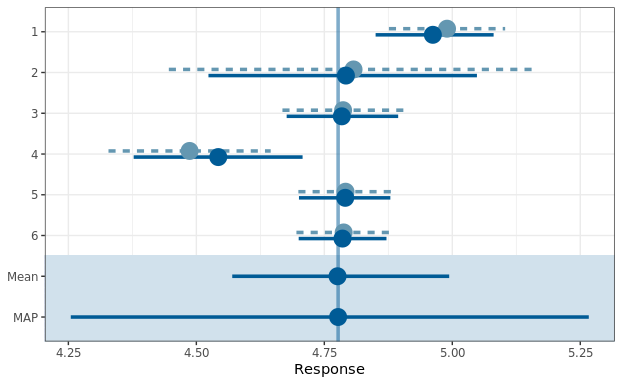In reference  the correct $$\Gamma$$ likelihood is used in contrast to the approximate normal approach above. Still, the results match very close, even for the outer quantiles. # MAP prior for the sampling standard deviation While the MAP analysis is performed for the $$\log$$ variance, we are actually interested in the MAP of the respective sampling standard deviation. Since the sampling standard deviation is a strictly positive quantity it is suitable to approximate the MCMC posterior of the MAP prior using a mixture of $$\Gamma$$ variates, which can be done using RBesT as: map_mc_post <- as.matrix(map_mc) sd_trans <- compose(sqrt, exp) mcmc_intervals(map_mc_post, regex_pars="theta", transformation=sd_trans)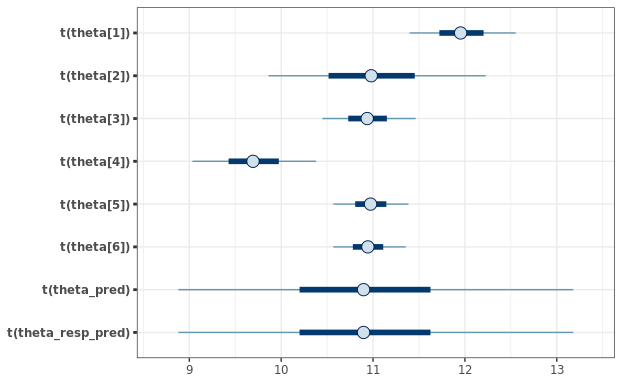map_sigma_mc <- sd_trans(map_mc_post[,c("theta_pred")]) map_sigma <- automixfit(map_sigma_mc, type="gamma") plot(map_sigma)$mix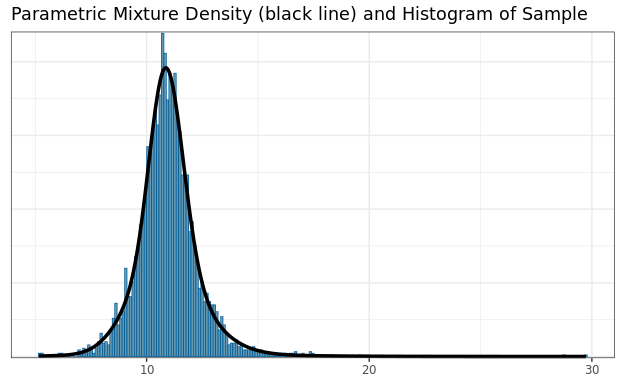## 95% interval MAP for the sampling standard deviation
summary(map_sigma)
##      mean        sd      2.5%     50.0%     97.5%
## 10.962936  1.393477  8.338368 10.903531 13.966982

# Normal approximation of a $$\log\Gamma$$ variate

For a $$\Gamma(y|\alpha, \beta)$$ variate $$y$$, which is $$\log$$ transformed, $$z = \log(y)$$, we have by the law of transformations for univariate densities:

$y|\alpha,\beta \sim \Gamma(\alpha,\beta)$ $p(z) = p(y) \, y = p(\exp(z)) \, \exp(z)$ $z|\alpha,\beta \sim \log\Gamma(\alpha,\beta)$ $\Leftrightarrow \exp(z)|\alpha,\beta \sim \Gamma(\alpha,\beta) \, \exp(z)$

The first and second moment of $$z$$ is then $E[z] = \psi(\alpha) - \log(\beta)$ $Var[z] = \psi^{(1)}(\alpha).$

A short simulation demonstrates the above results:

gamma_dist <- mixgamma(c(1, 18, 6))

## logGamma density
dlogGamma <- function(z, a, b, log=FALSE) {
n <- exp(z)
if(!log) {
return(dgamma(n, a, b) * n)
} else {
return(dgamma(n, a, b, log=TRUE) + z)
}
}

a <- gamma_dist[2,1]
b <- gamma_dist[3,1]
m <- digamma(a) - log(b)
v <- psigamma(a,1)

## compare simulated histogram of log transformed Gamma variates to
## analytic density and approximate normal
sim <- rmix(gamma_dist, 1E5)
mcmc_hist(data.frame(logGamma=log(sim)), freq=FALSE, binwidth=0.1) +
overlay_function(fun=dlogGamma, args=list(a=a,b=b), aes(linetype="LogGamma")) +
overlay_function(fun=dnorm, args=list(mean=m, sd=sqrt(v)), aes(linetype="NormalApprox"))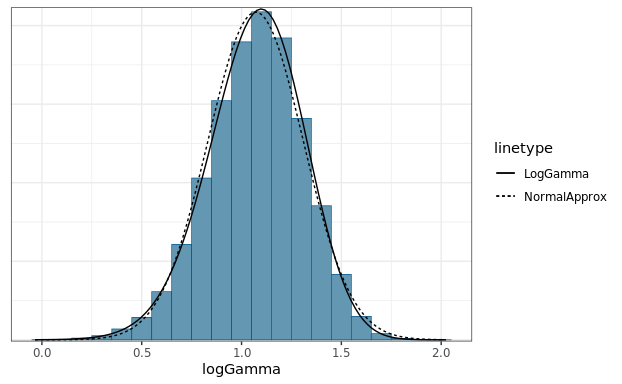We see that for $$\nu=9$$ only, the approximation with a normal density is reasonable. However, by comparing as a function of $$\nu$$ the $$2.5$$%, $$50$$% and $$97.5$$% quantiles of the correct distribution with the respective approximate distribution we can assess the adequatness of the approximation. The respective R code is accessible via the vignette overview page while here the graphical result is presented for two different $$\sigma$$ values: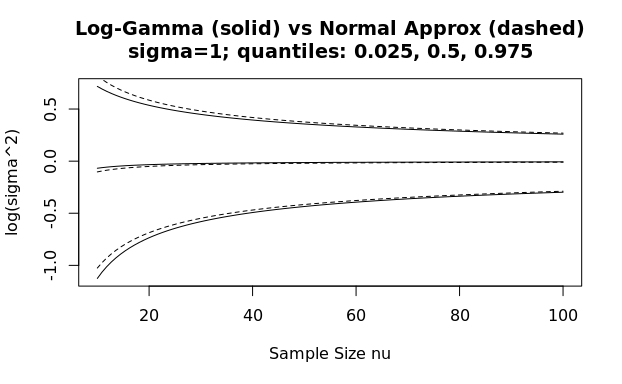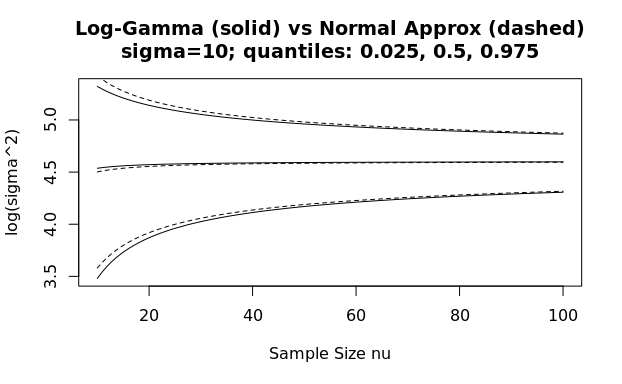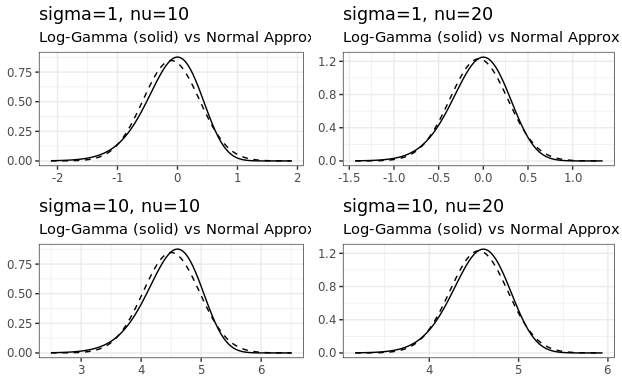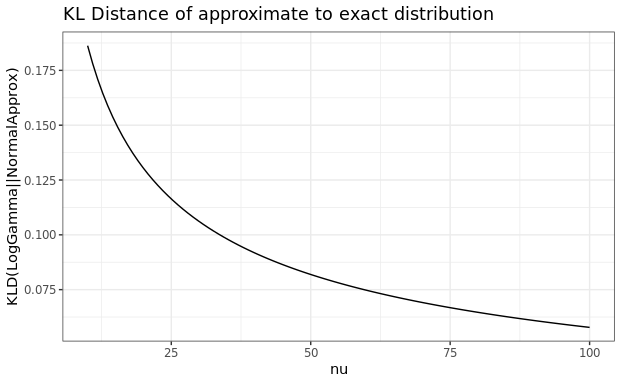## Acknowledgements

Many thanks to Ping Chen and Simon Wandel for pointing out an issue with the transformation as used earlier in this vignette.

## R Session Info

sessionInfo()
## R version 4.1.0 (2021-05-18)
## Platform: x86_64-pc-linux-gnu (64-bit)
## Running under: Ubuntu 20.04.4 LTS
##
## Matrix products: default
## BLAS:   /usr/lib/x86_64-linux-gnu/blas/libblas.so.3.9.0
## LAPACK: /usr/lib/x86_64-linux-gnu/lapack/liblapack.so.3.9.0
##
## locale:
##   LC_CTYPE=en_US.UTF-8       LC_NUMERIC=C
##   LC_TIME=en_US.UTF-8        LC_COLLATE=en_US.UTF-8
##   LC_MONETARY=en_US.UTF-8    LC_MESSAGES=en_US.UTF-8
##   LC_PAPER=en_US.UTF-8       LC_NAME=C
##  LC_MEASUREMENT=en_US.UTF-8 LC_IDENTIFICATION=C
##
## attached base packages:
##  stats     graphics  grDevices utils     datasets  methods   base
##
## other attached packages:
##  scales_1.1.1    purrr_0.3.4     bayesplot_1.8.1 tidyr_1.1.3
##  dplyr_1.0.8     ggplot2_3.3.5   RBesT_1.6-4     knitr_1.33
##
## loaded via a namespace (and not attached):
##   Rcpp_1.0.7           mvtnorm_1.1-2        prettyunits_1.1.1
##   ps_1.6.0             assertthat_0.2.1     digest_0.6.29
##   utf8_1.2.2           V8_3.4.2             plyr_1.8.6
##  R6_2.5.1             ggridges_0.5.3       backports_1.2.1
##  stats4_4.1.0         evaluate_0.14        highr_0.9
##  pillar_1.6.2         rlang_1.0.1          curl_4.3.2
##  callr_3.7.0          jquerylib_0.1.4      checkmate_2.0.0
##  rmarkdown_2.11       labeling_0.4.2       stringr_1.4.0
##  loo_2.4.1            munsell_0.5.0        compiler_4.1.0
##  xfun_0.25            rstan_2.21.2         pkgconfig_2.0.3
##  pkgbuild_1.2.0       rstantools_2.1.1     htmltools_0.5.2
##  tidyselect_1.1.1     tibble_3.1.3         gridExtra_2.3
##  codetools_0.2-18     matrixStats_0.60.1   fansi_0.5.0
##  crayon_1.4.2         withr_2.4.3          grid_4.1.0
##  jsonlite_1.7.2       gtable_0.3.0         lifecycle_1.0.1
##  sass_0.4.0